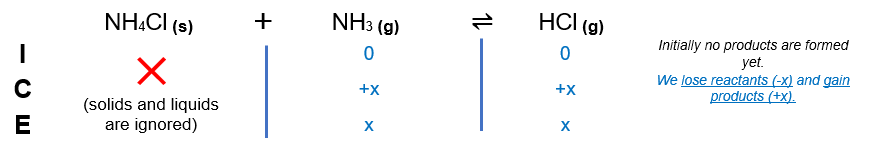# Problem: A sample of solid ammonium chloride was placed in an evacuated chamber and then heated, causing it to decompose according to the following reaction:NH4Cl(s) ⇌ NH3(g) + HCl(g)In a particular experiment, the equilibrium partial pressure of NH 3(g) in the container was 2.9 atm. Calculate the value of Kp for the decomposition of NH4Cl(s) at this temperature.

###### FREE Expert Solution

We are asked to calculate the value of Kp for the decomposition of NH4Cl(s) at this temperature.

Setup ICE chart:At equilibrium: PNH3 = 2.9 atm = x###### Problem Details

A sample of solid ammonium chloride was placed in an evacuated chamber and then heated, causing it to decompose according to the following reaction:

NH4Cl(s) ⇌ NH3(g) + HCl(g)

In a particular experiment, the equilibrium partial pressure of NH 3(g) in the container was 2.9 atm. Calculate the value of Kp for the decomposition of NH4Cl(s) at this temperature.